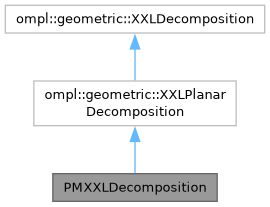PMXXLDecomposition Class Reference
Inheritance diagram for PMXXLDecomposition:[legend]

## Public Member Functions

PMXXLDecomposition (ompl::base::SpaceInformationPtr si, const PlanarManipulator *manip, const ompl::base::RealVectorBounds &xyBounds, const std::vector< int > &xySlices, const int thetaSlices, std::vector< int > &projectedJoints, bool diagonalEdges)
Constructor. The end of the links in projectedJoints constitute the different layers to this decomposition.

virtual int numLayers () const
Return the number of layers possible in this decomposition. Must be at least 1.

virtual bool steerToRegion (int r, int layer, const ompl::base::State *start, std::vector< ompl::base::State * > &states) const

virtual bool sampleFromRegion (int r, ompl::base::State *s, const ompl::base::State *seed=nullptr) const
Sample a valid state s from region r in layer 0.

void initializePartialManipulator (int layer, const double *seedAngles) const

void sampleEndEffectorPose (int region, Eigen::Affine2d &pose) const

void initializePartialSeed (int layer, const double *seedAngles, std::vector< double > &partialSeed) const

bool sampleRemainingJoints (int layer, ompl::base::State *s, const double *const seedVals, const std::vector< double > &partialSln) const

virtual bool sampleFromRegion (int r, ompl::base::State *s, const ompl::base::State *seed, int layer) const
Sample a state s from region r in the given layer.

virtual void project (const ompl::base::State *s, std::vector< double > &coord, int layer=0) const
Project the given State into the XXLDecomposition.

virtual void project (const ompl::base::State *s, std::vector< int > &layers) const
Project the state into the decomposition and retrieve the region for all valid layers.Public Member Functions inherited from ompl::geometric::XXLPlanarDecomposition
XXLPlanarDecomposition (const base::RealVectorBounds &xyBounds, const std::vector< int > &xySlices, const int thetaSlices, bool diagonalEdges=false)

XXLPlanarDecomposition (const base::RealVectorBounds &xyBounds, const std::vector< int > &xySlices, const int thetaSlices, double thetaLowerBound, double thetaUpperBound, bool diagonalEdges=false)

virtual int getNumRegions () const
Return the total number of regions in this decomposition.

virtual int getDimension () const
Return the dimension of this HiLoDecomposition.

virtual int locateRegion (const base::State *s) const
Return the id of the region that this state falls in.

virtual int locateRegion (const std::vector< double > &coord) const
Return the id of the region that this coordinate falls in.

virtual void getNeighbors (int rid, std::vector< int > &neighbors) const
Stores the given region's neighbors into a given vector.

virtual void getNeighborhood (int rid, std::vector< int > &neighborhood) const
Stores the given region's neighbors into the vector. This returns the 8-connected grid neighbors of the cell, regardless of whether diagonal edges exist.

virtual double distanceHeuristic (int r1, int r2) const
An admissible and consistent distance heuristic between two regions. Manhattan distance on grid.

void ridToGridCell (int rid, std::vector< int > &cell) const

int gridCellToRid (const std::vector< int > &cell) const
Return the region id corresponding to the (discrete) grid cell coordinates.

int coordToRegion (const std::vector< double > &coord) const
Return the region id of the given coordinate in the decomposition.

int coordToRegion (const double *coord) const

bool hasDiagonalEdges () const
Return true if the decomposition has diagonal edges.Public Member Functions inherited from ompl::geometric::XXLDecomposition
XXLDecomposition ()
Constructor.

virtual bool canSteer () const
Returns true if the method steerToRegion is available.

## Static Public Member Functions

static void frameToPose (const Eigen::Affine2d &frame, Eigen::VectorXd &pose)

## Protected Attributes

ompl::base::SpaceInformationPtr si_

const PlanarManipulatormanip_

std::vector< PlanarManipulatorpartialManips_

std::vector< int > projectedJoints_Protected Attributes inherited from ompl::geometric::XXLPlanarDecomposition
bool diagonalEdges_

base::RealVectorBounds xyBounds_

double thetaLow_ {-boost::math::constants::pi<double>()}

double thetaHigh_ {boost::math::constants::pi<double>()}

std::vector< int > xySlices_

int thetaSlices_

int numRegions_

double dx_

double dy_

double xSize_

double ySize_

double dTheta_

double thetaSize_

int dimension_

ompl::RNG rng_Protected Member Functions inherited from ompl::geometric::XXLPlanarDecomposition
void constructGraph ()

void getNonDiagonalNeighbors (int rid, std::vector< int > &neighbors) const

void getDiagonalNeighbors (int rid, std::vector< int > &neighbors) const

void sampleCoordinateFromRegion (int r, std::vector< double > &coord) const

void sampleCoordinateFromRegion (int r, double *coord) const

## Detailed Description

Definition at line 47 of file PlanarManipulatorXXLDecomposition.h.

The documentation for this class was generated from the following file: